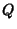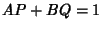## Bezout's Theorem

In general, two algebraic curves of degreesandintersect inpoints and cannot meet in more thanpoints unless they have a component in common (i.e., the equations defining them have a common factor). This can also be stated: ifandare two Polynomials with no roots in common, then there exist two other Polynomialsandsuch that. Similarly, givenPolynomial equations of degrees,, ...invariables, there are in generalcommon solutions.